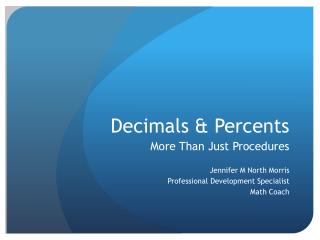Download PresentationDecimals & Percents

# Decimals & Percents

Download Presentation## Decimals & Percents

- - - - - - - - - - - - - - - - - - - - - - - - - - - E N D - - - - - - - - - - - - - - - - - - - - - - - - - - -
##### Presentation Transcript

1. Decimals & Percents More Than Just Procedures Jennifer M North Morris Professional Development Specialist Math Coach

2. Essential Question What types of lessons and learning experiences can increase the conceptual understanding of percent and decimals for students?

3. Objectives • Explore and engage in lessons which promote student thinking. • Collaboratewith other teachers to create a collection of learning experiences that will develop conceptual understanding.

4. Agenda: Decimals & Percents • Day 1: What is Conceptual Understanding? • Day 2: Where is the Decimal Fraction? • Day 3: Parts of a Whole • Day 4: Embracing Common Core

5. Move it!!

6. Introductions • Someone You Look Up To • Meet anyone (alive or passed) • Inspirational Educator (not in this room) • Take any of these people on vacation. Who? Where?

7. 24 x 16

8. 1.5 x 34

9. Number Talks Purpose: Develop conceptual understanding of mathematics • Make sense of the math • Learn from others • Get students Thinking!!

10. Number Talks: Key Ideas • Solve mentally (no paper) • Find several ways to solve the task • Silent thumbs up when finished • Get possible answers • Students share methods of solution • Teacher visually demonstrates student thinking as instructed by the student

11. Number Talk Example • http://www.schooltube.com/video/e26276ebb66a448486c9/

12. Number Talks: Why?

13. Standards for Mathematical Practice • Make sense of problems and persevere in solving them • Reason abstractly and quantitatively • Construct viable arguments and critique the reasoning of others • Model with mathematics • Use appropriate tools strategically • Attend to precision • Look for and make use of structure • Look for and express regularity in repeated reasoning

14. New Carpet! MsRouintree needs new carpet for her classroom. The room is a rectangle and measures 11.1 meters by 14.3 meters. How much carpeting does she need to order? Use numbers & a diagram

15. Conceptual Ideas

16. Applying Concepts

17. When do we divide by a decimal?

18. Dividing by Decimals • Create a scenario. • Solve with numbers and a diagram • Prior Knowledge? • How do we develop conceptual ideas?

19. Modeling: Circles • I have one pizza and want to give 1/5 slices. How many people can I share with? • 3 pizzas? • What is the expression?

20. Modeling: Circles • You have \$1.50 and want to buy gumballs that cost \$0.25. How many can you buy? • \$4.50? • What is the expression?

21. Modeling: Circles • 3.5 ÷ 0.5 • How do you model? • What is the Problem?

22. Modeling: Circles • 5.4 ÷ 0.2 • How do you model? • What is the Problem?

23. Modeling: Circles • 1.5 ÷ 0.25 • How do you model? • What is the Problem?

24. Where Would You Go From Here? Where do we need to go? How do we get there? Regularity?

26. Why Does this Work? • 49.92/15.6 • 499.2/156 • 4992/1560 • 4.992/1.56 • 0.4992/0.156

27. Where Would You Go From Here? Where do we need to go? How do we get there? Regularity?

28. Conceptual Understanding • Explore and engage in lessons which promote student thinking. • Collaboratewith other teachers to create a collection of lessons that will develop conceptual understanding.

29. Homework ?#\$@?!& Reflection • Journal (?) of where we see/use percent & decimals everyday • Reflection

30. Decimals & Percent More Than Just Procedures Jennifer M North Morris Professional Development Specialist Math Coach

31. Essential Question What types of lessons and learning experiences can increase the conceptual understanding of percents and decimals for students?

32. Objectives • Explore and engage in lessons which promote student thinking. • Collaboratewith other teachers to create a collection of lessons that will develop conceptual understanding.

33. Agenda: Decimals & Percents • Day 1: What is Conceptual Understanding? • Day 2: Where is the Decimal Fraction? Modeling with Base Ten Blocks • Day 3: Parts of a Whole • Day 4: Embracing the Common Core

34. Count the Dots

35. ⦁⦁⦁⦁⦁⦁ ⦁⦁⦁⦁⦁ ⦁⦁⦁⦁⦁⦁

36. 4.5 ÷ 1.5

37. Number Talks: Examples

38. Line it Up!

39. Calculator Reset Press # Select 3 (All) Press = (Yes)

40. Line It Up!!! Press T Select 2 (Line0) Press `=

41. Introductions • First educational memory • First mathematics memory • Favorite teacher • Why teach? Why math?

43. Other Ideas for Random Numbers? Less than? Greater than? Odd/Even Math Facts Practice 50-50 Chance! Choose Groups Other ideas…

44. Homework ?#\$@?! • Journal (?) of where we see/use percent & decimals everyday • Did you try anything new? • Reflections

45. Ordering Decimals Which of the two numbers is larger? Be ready to explain your reasoning.

46. Rules for Size of Whole Numbers • The number that has more digits is larger. • If both numbers have the same number of digits or columns, start comparing from the left. The first one that has a higher digit is higher.

47. Rules for Size of Decimals

48. Cash for Gold!!! 1.74 g 0.07 g 0.3 g

49. How Far? 1.3 miles this morning 3.09 miles at lunch 0.82 miles this afternoon

50. Book Fair! I have \$5 and buy a book that costs \$2.25. Do I have enough for the poster?